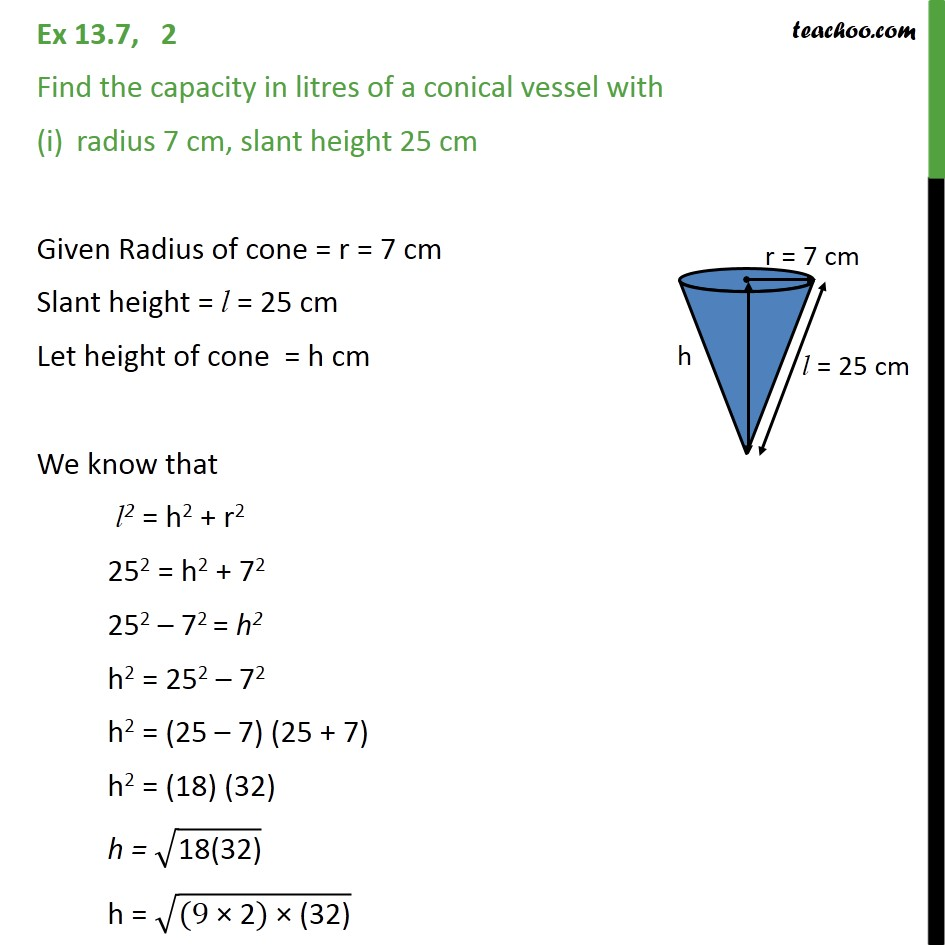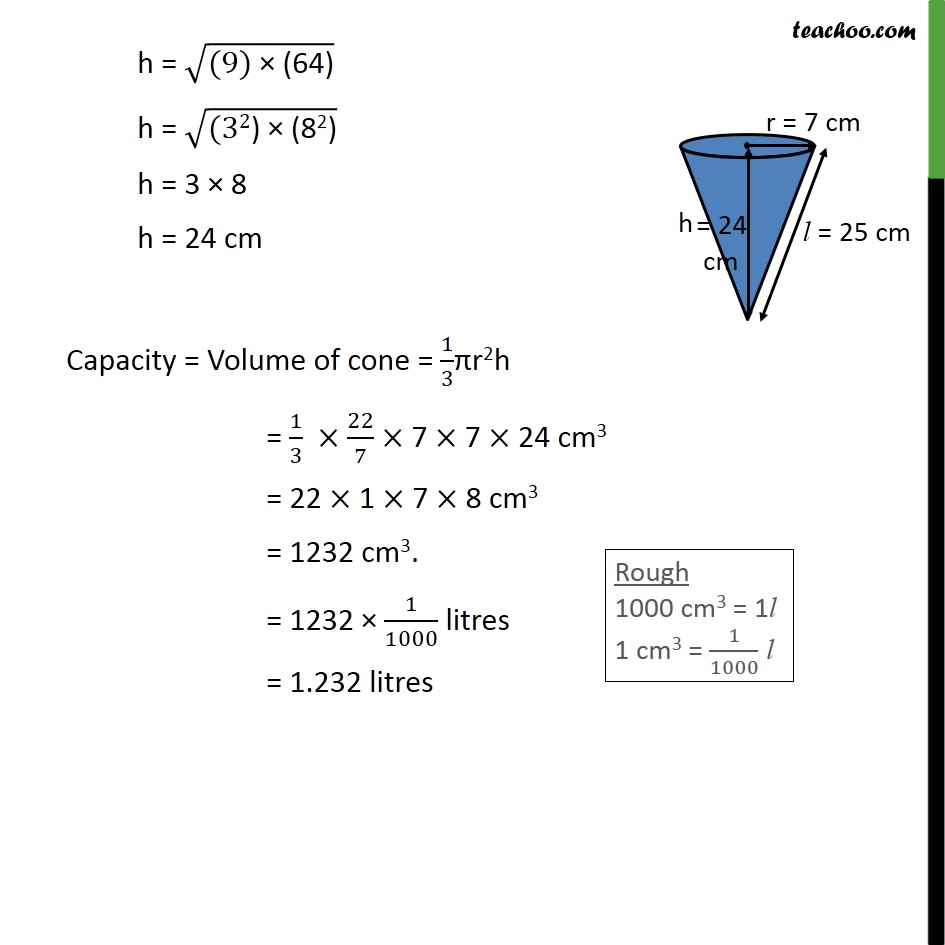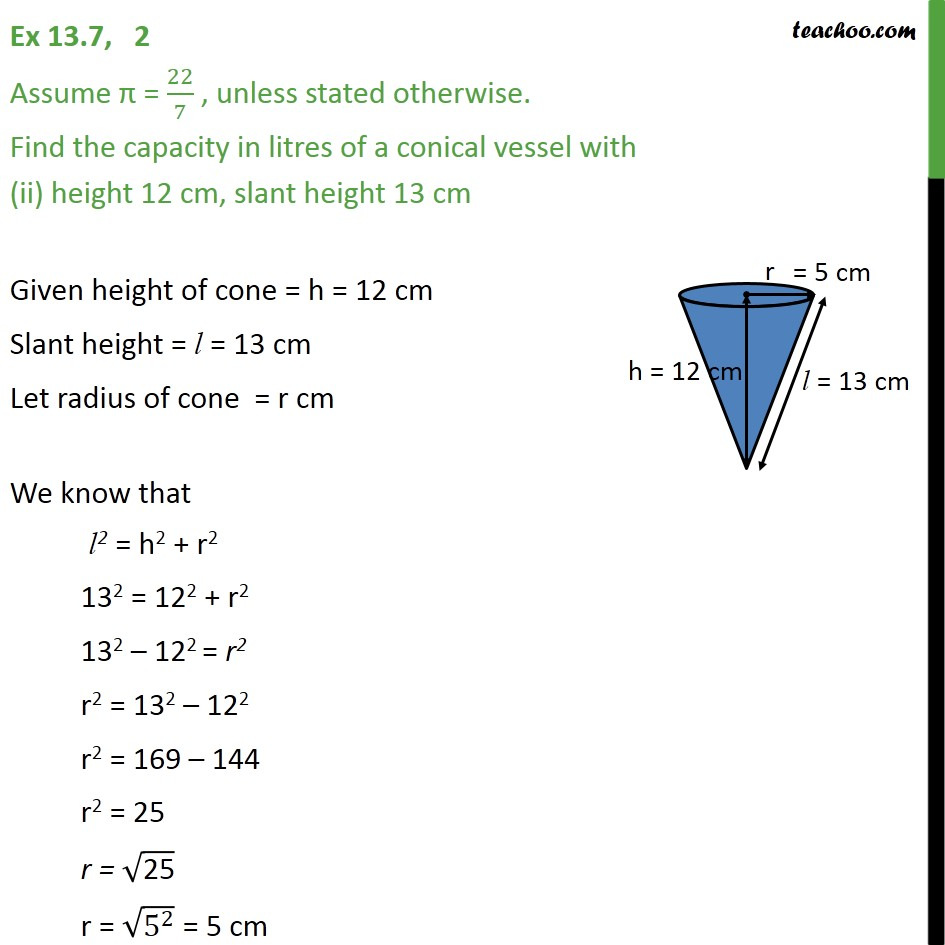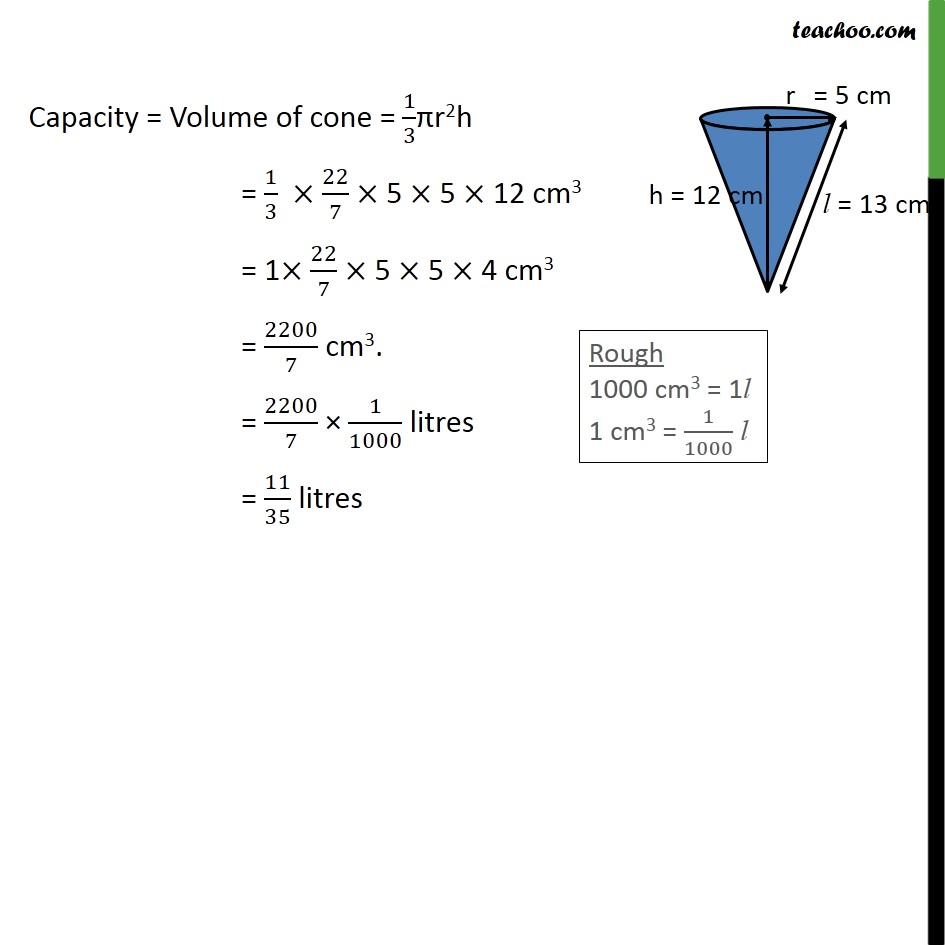Subscribe to our Youtube Channel - https://you.tube/teachoo

1. Chapter 13 Class 9 Surface Areas and Volumes
2. Serial order wise
3. Ex 13.7

Transcript

Ex 13.7, 2 Find the capacity in litres of a conical vessel with radius 7 cm, slant height 25 cm Given Radius of cone = r = 7 cm Slant height = l = 25 cm Let height of cone = h cm We know that l2 = h2 + r2 252 = h2 + 72 252 – 72 = h2 h2 = 252 – 72 h2 = (25 – 7) (25 + 7) h2 = (18) (32) h = √("18(32)" ) h = √("(9 × 2) × (32)" ) h = √("(9) × (64)" ) h = √("(32) × (82)" ) h = 3 × 8 h = 24 cm Capacity = Volume of cone = 1/3πr2h = 1/3 × 22/7 × 7 × 7 × 24 cm3 = 22 × 1 × 7 × 8 cm3 = 1232 cm3. = 1232 × 1/1000 litres = 1.232 litres Ex 13.7, 2 Assume π = 22/7 , unless stated otherwise. Find the capacity in litres of a conical vessel with (ii) height 12 cm, slant height 13 cm Given height of cone = h = 12 cm Slant height = l = 13 cm Let radius of cone = r cm We know that l2 = h2 + r2 132 = 122 + r2 132 – 122 = r2 r2 = 132 – 122 r2 = 169 – 144 r2 = 25 r = √("25" ) r = √(5^2 ) = 5 cm Capacity = Volume of cone = 1/3πr2h = 1/3 × 22/7 × 5 × 5 × 12 cm3 = 1× 22/7 × 5 × 5 × 4 cm3 = 2200/7 cm3. = 2200/7 × 1/1000 litres = 11/35 litres

Ex 13.7